Example Questions

← Previous 1 3

Example Question #1 : Equations / Solution Sets

Factor the following equation.

x– 16

(x)(x – 4)

(x + 4)(x + 4)

(x – 4)(x – 4)

(x + 4)(x – 4)

(x2)(4 – 2)

(x + 4)(x – 4)

Explanation:

The correct answer is (x + 4)(x – 4)

We neen to factor x– 16 to solve. We know that each parenthesis will contain an x to make the x2. We know that the root of 16 is 4 and since it is negative and no value of x is present we can tell that one 4 must be positive and the other negative. If we work it from the multiple choice answers we will see that when multiplying it out we get x+ 4x – 4x – 16. 4x – 4x cancels out and we are left with our answer.

Example Question #2 : Factoring Equations

If x3 – y3 = 30, and x2 + xy + y2 = 6, then what is x2 – 2xy + y2?

180

24

25

5

cannot be determined

25

Explanation:

First, let's factor x3 – y3 using the formula for difference of cubes.

x3 – y= (x – y)(x2 + xy + y2)

We are told that x2 + xy + y2 = 6. Thus, we can substitute 6 into the above equation and solve for x – y.

(x - y)(6) = 30.

Divide both sides by 6.

x – y = 5.

The original questions asks us to find x2 – 2xy + y2. Notice that if we factor x2 – 2xy + y2 using the formula for perfect squares, we obtain the following:

x2 – 2xy + y= (x – y)2.

Since we know that (x – y) = 5, (x – y)2 must equal 52, or 25.

Thus, x2 – 2xy + y= 25.

Example Question #3 : Factoring Equations

if x – y = 4 and x2 – y = 34, what is x?

9

10

15

12

6

6

Explanation:

This can be solved by substitution and factoring.

x2 – y = 34 can be written as y = x2 – 34 and substituted into the other equation: x – y = 4 which leads to x – x2 + 34 = 4 which can be written as x2 – x – 30 = 0.

x2 – x – 30 = 0 can be factored to (x – 6)(x + 5) = 0 so x = 6 and –5 and because only 6 is a possible answer, it is the correct choice.

Example Question #2 : Equations / Inequalities

If x2 + 2ax + 81 = 0.  When a = 9, what is the value of x?

–18

9

–9

0

3

–9

Explanation:

When a = 9, then x2 + 2ax + 81 = 0 becomes

x2 + 18x + 81 = 0.

This equation can be factored as (x + 9)2 = 0.

Therefore when a = 9, x = –9.

Example Question #5 : How To Factor An Equation

If f(x) has roots at x = –1, 0 and 2, which of the following could be the equation for f(x)?

f(x) = x– x+ 2x

f(x) = x– x– 2x

f(x) = x4 + x3 – 2x2

f(x) = x– x – 2

f(x) = x2 + x – 2

f(x) = x– x– 2x

Explanation:

In general, if a function has a root at x = r, then (x – r) must be a factor of f(x). In this problem, we are told that f(x) has roots at –1, 0 and 2. This means that the following are all factors of f(x):

(x – (–1)) = x + 1

(x – 0) = x

and (x – 2).

This means that we must look for an equation for f(x) that has the factors (+ 1), x, and (x – 2).

We can immediately eliminate the function f(x) = x2 + x – 2, because we cannot factor an x out of this polynomial. For the same reason, we can eliminate f(x) = x– x – 2.

Let's look at the function f(x) = x– x+ 2x. When we factor this, we are left with x(x2 – x + 2). We cannot factor this polynomial any further. Thus, x + 1 and x – 2 are not factors of this function, so it can't be the answer.

Next, let's examine f(x) = x4 + x3 – 2x.

We can factor out x2.

x(x+ x – 2)

When we factor x+ x – 2, we will get (x + 2)(x – 1). These factors are not the same as x – 2 and x + 1.

The only function with the right factors is f(x) = x– x– 2x.

When we factor out an x, we get (x– x – 2), which then factors into (x – 2)(+ 1). Thus, this function has all of the factors we need.

The answer is f(x) = x– x– 2x.

Example Question #2 : How To Factor An Equation

Factor 36x2 – 49y2.

(6x + 7y)(6x – 7y)

cannot be factored

(6x – 7y)(6x – 7y)

6x2 – 7y2

(6x + 7y)(6x + 7y)

(6x + 7y)(6x – 7y)

Explanation:

This is a difference of squares. The difference of squares formula is a2 – b2 = (a + b)(a – b). In this problem, a = 6x and b = 7y.

So 36x2 – 49y= (6x + 7y)(6x – 7y).

Example Question #7 : How To Factor An Equation

Solve for x: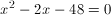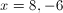Explanation:

Find two numbers that add to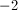and multiply to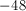Factors of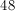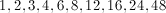You can use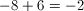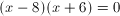Then make each factor equal 0.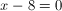and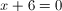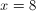and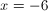Example Question #8 : How To Factor An Equation

Find the roots of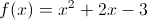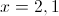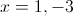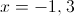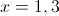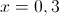Explanation:

Factoring yields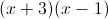giving roots of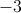and.

Example Question #9 : How To Factor An Equation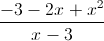Find the root of the equation above.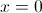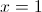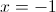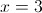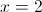Explanation:

The numerator can be factored into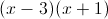.

Therefore, it can cancel with the denominator. So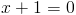imples.

Example Question #4 : Factoring Equations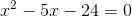Solve for.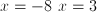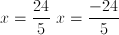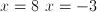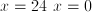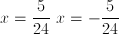Explanation:Find all factors of 24

1, 2, 3,4, 6, 8, 12, 24

Now find two factors that add up to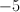and multiply to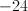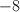and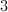are the two factors.

By factoring, you can set the equation to be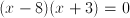If you FOIL it out, it gives you.

Set each part of the equation equal to 0, and solve for.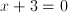and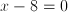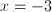and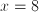← Previous 1 3